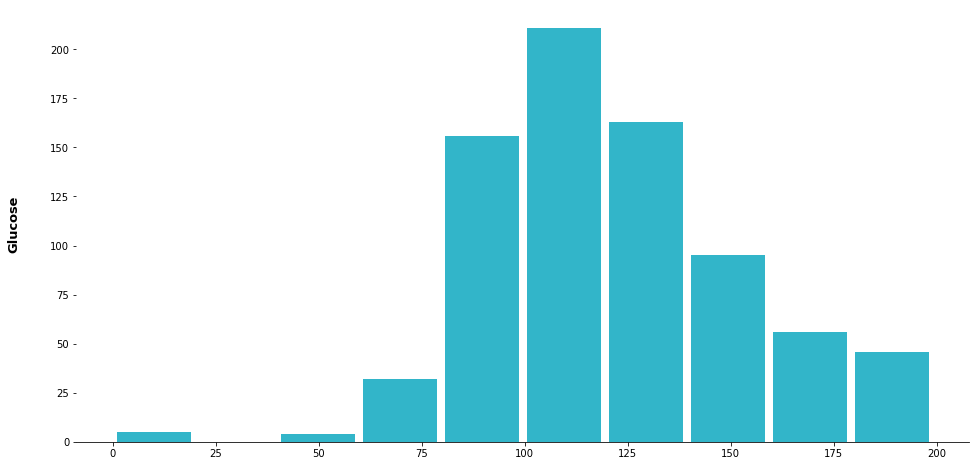Picture by XPS, Unsplash.

During the Exploratory Data Analysis or EDA stage one of the key things you’ll want to do is understand the statistical distribution of your data. Histograms are one of the quickest and easiest way to achieve this, since they group together numeric data into bins to provide a simplified representation of the data.

The functionality for plotting them is built directly into Pandas. However, technically, the histograms and other data visualisations in Pandas aren’t actually produced by Pandas itself. Instead, Pandas provides a wrapper to the Matplotlib PyPlot library which gives you quick access to the main features of PyPlot, without the need to write all of the underlying code.

The out-of-the-box functionality for visualisations using the Pandas wrapper doesn’t allow you to do everything in a one-liner, but you can do most things, and you can easily add any missing functionality by utilising Matplotlib code on top of the Pandas functions. Here’s how it’s done.

Most of the things we’re looking at here can be done using solely the Pandas library. However, as we may need to call Matplotlib and Numpy, we’ll load these packages too.

``````import pandas as pd
import numpy as np
import matplotlib.pyplot as plt
``````

I’ve used the Pima Indians diabetes dataset here, as it contains a good mix of data. You can download this from various places, including the UCI Machine Learning Repository. It’s a good idea to tidy up the column names upon import and to drop the row of original column headers, which aren’t formatted nicely for use with Pandas.

``````df = pd.read_csv('diabetes.csv',
names=['pregnant','glucose','bp','skin_thickness',
'insulin','bmi','pedigree','age','outcome'],
skiprows=1)
``````
pregnant glucose bp skin_thickness insulin bmi pedigree age outcome
0 6 148 72 35 0 33.6 0.627 50 1
1 1 85 66 29 0 26.6 0.351 31 0
2 8 183 64 0 0 23.3 0.672 32 1
3 1 89 66 23 94 28.1 0.167 21 0
4 0 137 40 35 168 43.1 2.288 33 1

### Creating a histogram of a specific column

To create a histogram of a specific column or Series from our Pandas dataframe we can append the `.hist()` function after defining the dataframe and column name. By default, this gives us a histogram with a standard size and colour and no title, with data spread across 10 bins. This gives us a good view of where glucose levels lie within the data.

``````glucose = df.glucose.hist()
``````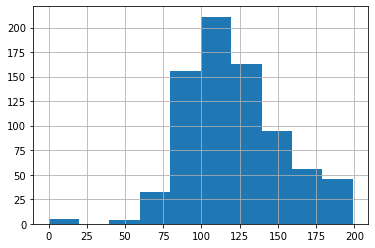#### Changing the histogram size

To change the size of the standard histogram we can add the `figsize()` argument and pass in a tuple of values. The first one is the width in inches (how quaint), and the second is the height in inches.

``````glucose = df.glucose.hist(figsize=(16,4))
``````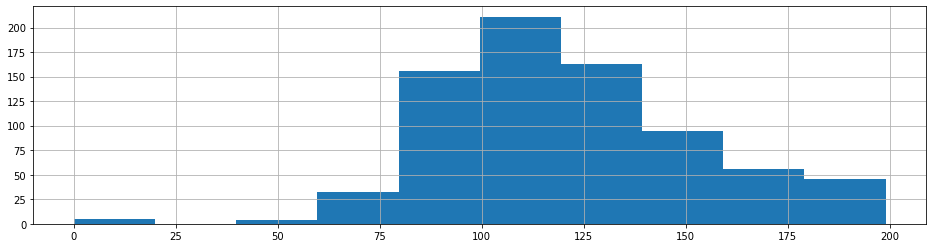#### Adding a title to the histogram

To add a title you can append `set_title()` and pass in a string value. There’s more you can do with titles, such as changing the size and weight of the font, but you need to do this using Matplotlib.

``````glucose = df.glucose.hist(figsize=(7.2,4)).set_title('Glucose')
``````

#### Changing the number of bins

The standard histogram defaults to 10 bins, however, you can change the number of bins by passing an integer to the `bins` argument of the `hist()` function. Setting bins to 100 gives a more granular view of the data.

``````glucose = df.glucose.hist(figsize=(7.2,4), bins=100).set_title('Glucose')
``````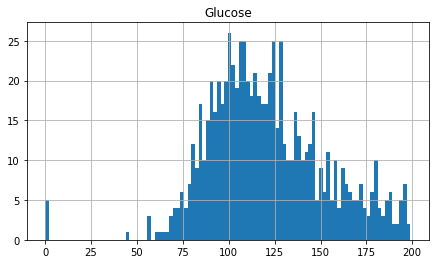#### Turning off the grid

If you want a more minimalist view of your data you can turn off the grid lines by passing in the `False` to the `grid` argument, which is set to `True` by default.

``````glucose = df.glucose.hist(figsize=(7.2,4), grid=False).set_title('Glucose')
``````#### Changing the colour of histograms

By default, Pandas histograms are dark blue, but you can define a custom colour by passing in a colour value. This can either be a named colour `red` or `orange`, or it can be a specific hex code, like `#32B5C9`.

``````glucose = df.glucose.hist(figsize=(7.2,4), color="orange").set_title('Glucose')
``````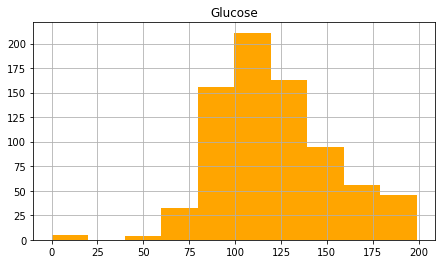``````age = df.age.hist(figsize=(7.2,4), color="#32B5C9").set_title('Age')
``````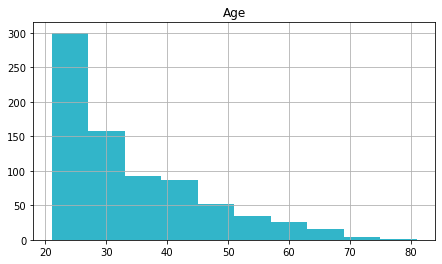#### Adding edge colours to histograms

If you want to make the distinction between each bar in your histogram a bit more distinct, you can pass in a colour value to the `ec` argument which changes the edge colour. Here’s the chart above with the grid turned off and the edge colour set to white.

``````age = df.age.hist(figsize=(7.2,4), color="#32B5C9", ec="white", grid=False).set_title('Age')
``````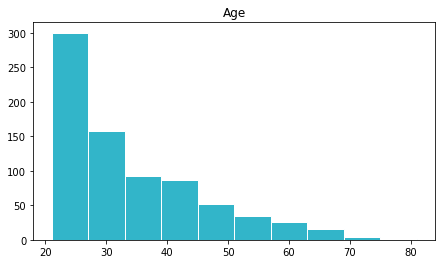#### Changing histogram orientation

By default, Pandas histograms are displayed vertically, but you can change this to horizontal by passing in the optional argument `orientation='horizontal'` to the `hist()` function.

``````glucose = df.glucose.hist(figsize=(7.2,4), orientation='horizontal').set_title('Glucose')
``````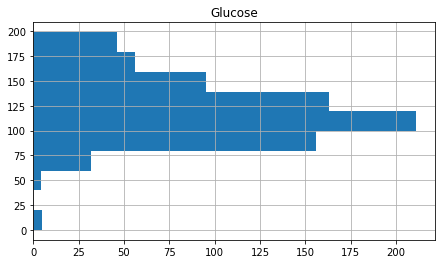### Creating histogram subplots

To view the statistical distributions of all of the numeric columns in your dataframe, instead of passing in a specific column, you can provide the entire dataframe. Pandas will automatically create a single chart with a subplot for each of the numeric columns. In the below example, I’ve manually defined the figure size so it fits the width of the page.

``````histograms = df.hist(figsize=(16,8))
``````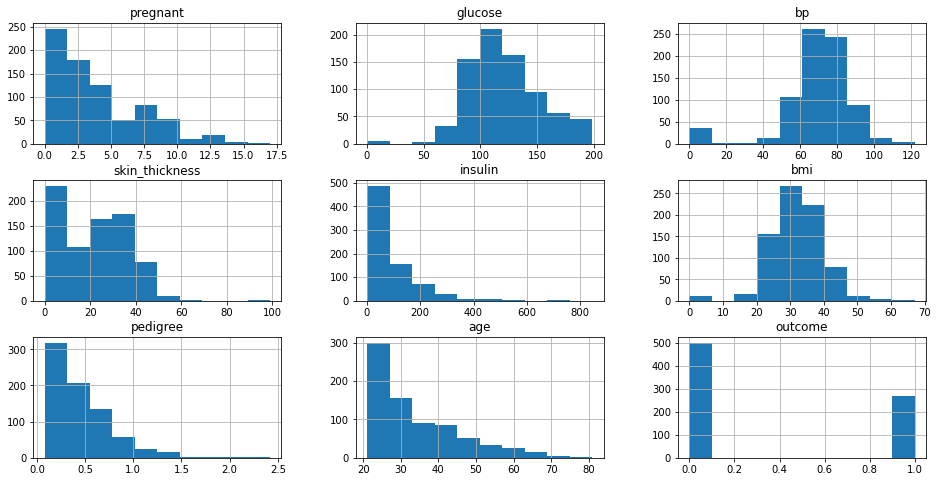#### Selecting specific data for subplots

If you want to view two or more specific histogram subplots of your numeric data you’ll need to bring in a little Matplotlib code. Firstly, we’ll use the `subplots()` function of PyPlot to create a figure containing 1 row and 2 columns, with a total size of 16 inches by 4 inches. Then we’ll create two histograms as we did above, but we’ll define which position they occupy on `ax` by passing in `axes` for the left column and `axes` for the right.

``````fig, axes = plt.subplots(1, 2, figsize=(16,4))

glucose = df.glucose.hist(ax=axes).set_title('Glucose')
outcome = df.age.hist(ax=axes).set_title('Diabetes')
``````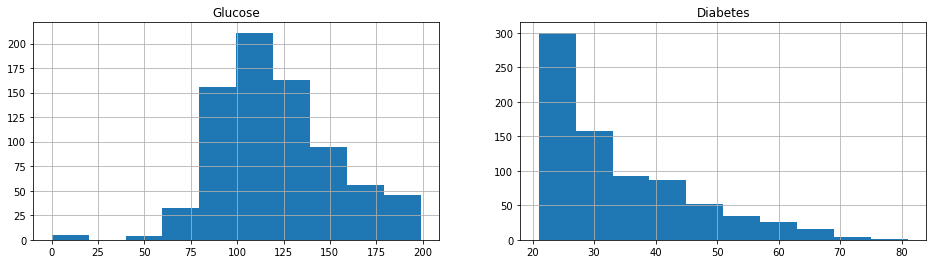It’s easy to repeat this process for the more than two histograms, of course. Simply change the number of subplots from `1,2` to `1,3` and add an additional histogram to be displayed in position `axes`.

``````fig, axes = plt.subplots(1, 3, figsize=(16,4))

age = df.age.hist(ax=axes).set_title('Age')
glucose = df.glucose.hist(ax=axes).set_title('Glucose')
outcome = df.age.hist(ax=axes).set_title('Diabetes')
``````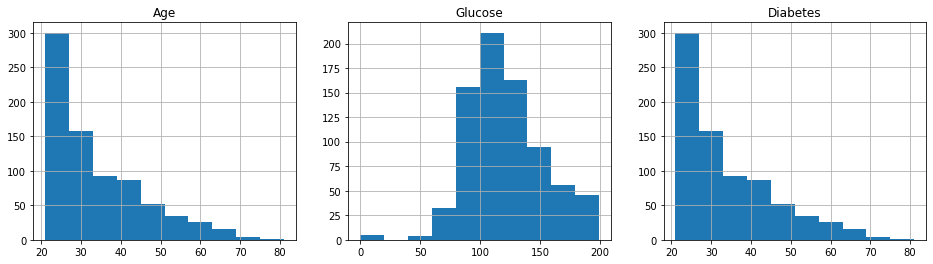### Creating stacked histograms

If you have two columns of data that share the same unit of measure, you can plot them on a stacked histogram by passing in the argument `stacked=True`. There’s no data like that in this dataset, so here’s a completely nonsensical example, just to show you what it looks like.

``````df[['glucose','insulin']].plot.hist(stacked=True, bins=10, figsize=(7.2,4))
``````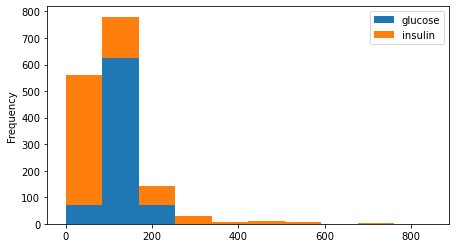You can combine some of the approaches above with some additional Matplotlib code to achieve some more stylish designs. In the example below I’ve added the `rwdith=0.9` argument to increase the width between the bars, and have passed in some extra arguments to hide the spines, remove the title, and add some larger axis labels.

``````ax = df.hist(column='glucose',
bins=10,
grid=False,
figsize=(16,8),
color="#32B5C9",
rwidth=0.9)

ax = ax
for x in ax:

x.set_title("")